Design Project 1

Due: Friday, March 6, 2009

# Introduction and Objectives

The objective of this project is to implement an efficient set of programs in MIPS assembly language using the SPIM simulator. The set of programs which are to be the "workload" for the computer are given below. Your objective is to convert these programs into MIPS and measure the performance of your conversions.

The following sections provide a discussion of the elements to be used for the performance analysis of your computer, the workload set of programs, guidance for what is required and how to accomplish it, and a description of what to turn in.

This project is to be an individual effort. You are allowed to discuss issues of a theoretical nature with each other, however NOT implementation details. You may specifically NOT share any code. Although the SPIM simulator supports macro assembly instructions you will be limited to instructions described in the back cover of your book.

Grading will be based on how well you meet the goals of the project, the performance of the workload as defined below, and on a typed report. All your design ideas should be presented in your report.

# Performance Evaluation

We will use the following equations to determine CPU time for the workload:

1.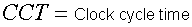2.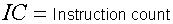3.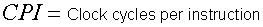4.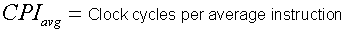5.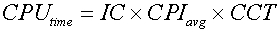6.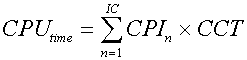You will measure the performance of the workload using equation 6. Remember that IC is not just the count of instructions on a printout, but rather the count of instructions that are executed. Because we are using a simulator we will define CCT as 1 ns.

Below are the CPI's for each instruction found in the back of the book:

 Instruction CPI All R-type 3 lw 6 sw 4 beq 2 bne 2 j 2 jr 2 jal 5 All other I-type 3

The workload consists of the following main program and test functions. These are being given to you in Java. You might want to modify the Java programs to help you count the number of instructions that are executed. Make sure you test the Java programs them, prior to converting to MIPS.

## Main

```public static void main(String[]  args) {
String string1 = "This project must be an individual effort. You  are "
+ "allowed to discuss issues of a theoretical nature with "
+ "each other, however NOT implementation details. Although "
+ "the SPIM simulator supports macro assembly instructions "
+ "you will be limited to instructions described in the back          "
String key1 = "book";
String key2 = "This";
String key3 = "individual";
String key4 = "constraints";

int Y1[] = {0,29,8,2,15,23,12,3,14,18,11,6,12};
int Y2[] = {7,2,3,8,79,9,10,4,7};
int Y3[] = {45,68,82,107,55,77};

int match;

sieve();
sieve();
sieve();

quickSort(Y1,13);
for(int i = 0; i<Y1.length; i++)
System.out.print("" + Y1[i] + " ");
System.out.println(" ");

quickSort(Y2,9);
for(int i = 0; i<Y2.length; i++)
System.out.print("" + Y2[i] + " ");
System.out.println(" ");

quickSort(Y3,6);
for(int i = 0; i<Y3.length; i++)
System.out.print("" + Y3[i] + " ");
System.out.println("\n");

match = stringMatch(string1,key1);
if(match == 1) System.out.println("match\n");

match = stringMatch(string1,key2);
if(match == 1) System.out.println("match\n");

match = stringMatch(string1,key3);
if(match == 1) System.out.println("match\n");

match= stringMatch(string1,key4);
if(match == 1) System.out.println("match\n");
} //main```

## Program 1 String Matching (Optional, 10 bonus points)

Program 1 you must write yourselves. The interface is given below. The requirements are that the function must determine whether key is contained in string. Adjust the main program if you don't do the string matching.

`public static int stringMatch (String testString, String key)          `

## Program 2 Sieve of Erastosthenes (Required)

Program 2 implements a method of finding prime numbers. This is an example of a computer performing arithmetic. This program works by creating an array of flags, and then setting the flag true if the number is prime and false if not.

```public static void sieve() {
int SIZE = 400;
int SQRT_SIZE = 20;

int[] prime = new int[SIZE+1];
int i, j;

prime = prime = 0; //0 and 1 are not prime
for(i=2; i<=SIZE; i++)
prime[i]= 1;           //all others may be prime

for(j=2; j<=SQRT_SIZE; j++) {      //the next loop excludes all multiples of j
for(i=2*j; i<=SIZE; i+=j)
prime[i] = 0;
} //for

for(int k=0; k<=SIZE; k++)
System.out.println(k + " " + prime[k]);
} //sieve```

## Program 3 Quick Sort (Optional, 10 bonus points)

Program 3 implements the quick sort algorithm, which sorts an array of numbers. Adjust the main program if you don't do the quick sort.

```public static void quickSort(int[] x, int n) {
qSort(x,0,n-1);
} //quickSort

public static void qSort(int x[],int left, int right) {
int middle;
if (left < right) {
middle = partition(x,left,right);
qSort(x,left,middle);
qSort(x,middle+1,right);
} //if
} //qSort

public static int partition(int x[], int left, int right) {
int pivot, l, r, temp;
pivot = x[left];
l = left-1;
r = right+1;
while (true) {
do {r--;} while (x[r] > pivot);
do {l++;} while (x[l] < pivot);
if (l < r) {
temp = x[l];
x[l] = x[r];
x[r]= temp;
} //if
else
return r;
} //while
} //partition ```

# Guidelines for Completing the Task

1. Get a copy of the SPIM simulator. It can be downloaded from http://www.cs.wisc.edu/~larus/spim.html. There you will also be able to find a complete description of the software. The SPIM simulator is available in the Haggerty computer labs.
2. Make sure the Java programs work correctly.
3. Review the appropriate chapters of the book.
4. Define how you will collect the performance data.
5. Convert your programs using the recommended steps.
1. Assign the variables to the registers.
2. Convert the body of the routine.
3. Add the code to save the appropriate registers to the stack and restore them.

You may modify these programs to improve their performance. Just make sure that the programs continue to function as originally designed.

# What To Turn In

You will be turning in a formal report. Follow the general directions for submitting your project. You should include at least the following sections. Here is the assessment form used for the project.

1. Introduction/Summary of the project – including whether the program was successfully tested, final performance numbers, and bonus eligibility.
2. Discussion of your design decisions and process (for example, what steps you went through to convert the Java program to MIPS).
3. Verification methods. The report must state whether the software works properly or not, and discuss the verification and testing methods used. All known bugs or implementation issues should be identified and discussed.
4. Performance analysis. The report must discuss and detail the methods used to count the total cycle count and simulated execution time, and give the results. Do this only on the sieve program.
5. High-level code for your program
6. MIPS/SPIM program code
7. What you learned. Discussion and conclusions.

# Instruction Efficiency Bonus (Maximum 10 points)

An efficiency bonus is available for the project, based on utilizing a smaller subset of the MIPS instruction set for full implementation (not counting syscall instructions).

To receive this bonus, you must successfully implement the workload using no more than the following set of instructions: ADD, ADDI, SUB, AND, OR, ORI, SLT, SRL, LW, SW, BEQ, LUI, JAL, JR.

# Minimum Instruction Bonus (Maximum 5 points)

The student turning in a successfully implemented and verified program using the smallest instruction set (fewest number of unique MIPS instructions used) will receive a 5 point bonus.

# Error Discovery Bonus (Maximum 5 points)

The student finding the most errors in the programs listed above or in a substantial instruction I've given. Only one student gets credit for each error. The one who reports the error first.